# How to Hide Axis Text Ticks or Tick Labels in Matplotlib?

• Last Updated : 15 Sep, 2022

The Matplotlib library by default shows the axis ticks and tick labels. Sometimes it is necessary to hide these axis ticks and tick labels. This article discusses some methods by which this can be done.

• Ticks: The axes’ points are marked with ticks, which are also known as little geometrical scale lines.
• Tick labels: They are the name given to the ticks. Alternately, we may say that tick labels are text-filled ticks known as Text Ticks.
• Axes: The X-axis and Y-axis are referred to as axis labels.

### Functions used:

• xticks(ticks=None, labels=None, **kwargs): used to get and set the current tick locations and labels of the x-axis.
• yticks(ticks=None, labels=None, **kwargs): used to get and set the current tick locations and labels of the y-axis.
• set_visible(boolean)- sets visibility

### Method 1: Select all columns except one by setting the tick labels to be empty

The functions xticks() and yticks() are used to denote positions using which a data point is supposed to be displayed. They take a list as an argument. Thus, axis text ticks or tick labels can be disabled by setting the xticks and yticks to an empty list as shown below:

```plt.xticks([])
plt.yticks([])```

## Python3

 `import` `matplotlib.pyplot as plt` `x1 ``=` `[``5``, ``8``, ``10``]``y1 ``=` `[``12``, ``16``, ``8``]``x2 ``=` `[``6``, ``9``, ``12``]``y2 ``=` `[``14``, ``10``, ``8``]` `plt.plot(x1, y1, ``'g'``, linewidth``=``7``)``plt.plot(x2, y2, ``'b'``, linewidth``=``7``)` `plt.title(``'Disabling xticks and yticks'``, fontsize``=``20``)` `plt.xlabel(``'X axis'``, fontsize``=``15``)``plt.ylabel(``'Y axis'``, fontsize``=``15``)` `# disabling xticks by Setting xticks to an empty list``plt.xticks([]) ` `# disabling yticks by setting yticks to an empty list``plt.yticks([]) ` `plt.show()`

Output: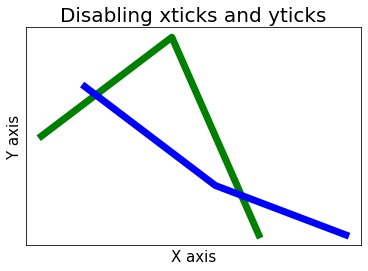### Method 2: Select all columns except one by setting the color white

By default, in the Matplotlib library, plots are plotted on a white background. Therefore, setting the color of tick labels as white can make the axis tick labels hidden. For this only color, the attribute needs to pass with w (represents white) as a value to xticks() and yticks() function. Implementation is given below:

## Python3

 `import` `matplotlib.pyplot as plt` `plt.plot([``5``, ``10``, ``20``], [``20``, ``10``, ``50``], color``=``'g'``)` `plt.xlabel(``"X Label"``)``plt.ylabel(``"Y Label"``)` `# xticks color white``plt.xticks(color``=``'w'``)` `# yticks color white``plt.yticks(color``=``'w'``)` `plt.show()`

Output: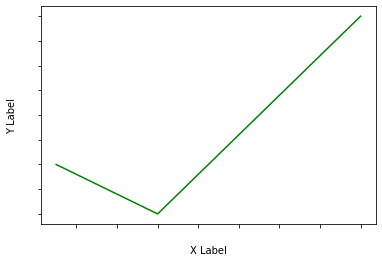### Method 3: Select all columns except one by using NullLocator()

A null Locator is a type of tick locator that makes the axis ticks and tick labels disappear. Simply passing NullLocator() function will be enough.

## Python3

 `import` `numpy as np``import` `matplotlib.ticker as ticker` `ax ``=` `plt.axes()` `x ``=` `np.random.rand(``100``)``ax.plot(x, color``=``'g'``)` `ax.xaxis.set_major_locator(ticker.NullLocator())``ax.yaxis.set_major_locator(ticker.NullLocator())`

Output: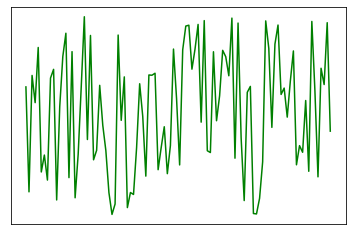### Method 4:Select all columns except one by placing blank space

Observe the syntax of xticks() and yticks() carefully, if even just a space is passed to them along with the data the output will be our desired result.

## Python3

 `import` `matplotlib.pyplot as plt` `x ``=` `[``5``, ``8``, ``15``, ``20``, ``30``]``y ``=` `[``15``, ``10``, ``8``, ``20``, ``15``]``plt.plot(x, y, color``=``'g'``, linewidth``=``5``)` `# x-label as blank``plt.xticks(x, ``" "``)` `# y-label as blank``plt.yticks(y, ``" "``)` `plt.show()`

Output: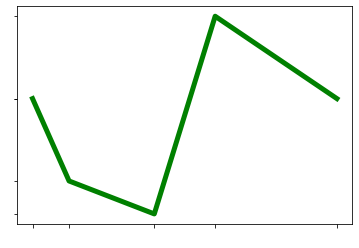### Method 5: Select all columns except one by assigning a label as space

This method is just a modification of method 4. Instead of passing an empty string, assign a label as space in the function itself.

## Python3

 `import` `matplotlib.pyplot as plt` `x ``=` `[``5``, ``8``, ``15``, ``20``, ``30``]``y ``=` `[``15``, ``10``, ``8``, ``20``, ``15``]``plt.plot(x, y, color``=``'g'``, linewidth``=``5``)` `plt.xticks(x, labels``=``" "``)``plt.yticks(y, labels``=``" "``)` `plt.show()`

Output: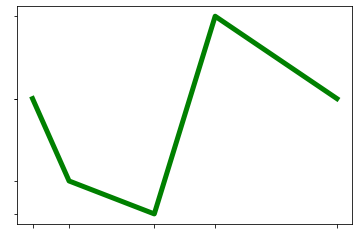### Method 6: Select all columns except one by setting set_visible(False).

Using set_visibile() we can also set the visibility of tick labels as False, which will not make them appear in our plot. This method hides labels as well as ticks, so if some requirement needs ticks to be displayed this isn’t the option, multiple methods shown above would stand ideal though.

## Python3

 `import` `matplotlib.pyplot as plt` `x ``=` `[``5``, ``8``, ``15``, ``20``, ``30``]``y ``=` `[``15``, ``10``, ``8``, ``20``, ``15``]``plt.plot(x, y, color``=``'g'``, linewidth``=``5``)` `# getting current axes``a ``=` `plt.gca()` `# set visibility of x-axis as False``xax ``=` `a.axes.get_xaxis()``xax ``=` `xax.set_visible(``False``)` `# set visibility of y-axis as False``yax ``=` `a.axes.get_yaxis()``yax ``=` `yax.set_visible(``False``)` `plt.show()`

Output: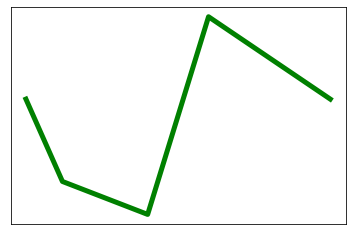My Personal Notes arrow_drop_up